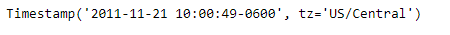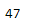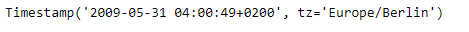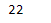# Python | Pandas Timestamp.weekofyear

• Last Updated : 08 Jan, 2019

Python is a great language for doing data analysis, primarily because of the fantastic ecosystem of data-centric python packages. Pandas is one of those packages and makes importing and analyzing data much easier.

Pandas` Timestamp.weekofyear` attribute return an integer value which is the ordinal value of the week in which the date of the given Timestamp object lies.

Syntax : Timestamp.weekofyear

Parameters : None

Return : week of year

Example #1: Use `Timestamp.weekofyear` attribute to find the ordinal value of the week in which the date of the given Timestamp object lies.

 `# importing pandas as pd ` `import` `pandas as pd ` ` `  `# Create the Timestamp object ` `ts ``=` `pd.Timestamp(year ``=` `2011``,  month ``=` `11``, day ``=` `21``,  ` `           ``hour ``=` `10``, second ``=` `49``, tz ``=` `'US/Central'``) ` ` `  `# Print the Timestamp object ` `print``(ts) `

Output :Now we will use the `Timestamp.weekofyear` attribute to find ordinal value of the week

 `# return the week number ` `ts.weekofyear `

Output :As we can see in the output, the `Timestamp.weekofyear` attribute has returned 47 indicating that the date in the give Timestamp object falls in the 47th week of the year.

Example #2: Use `Timestamp.weekofyear` attribute to find the ordinal value of the week in which the date of the given Timestamp object lies.

 `# importing pandas as pd ` `import` `pandas as pd ` ` `  `# Create the Timestamp object ` `ts ``=` `pd.Timestamp(year ``=` `2009``,  month ``=` `5``, day ``=` `31``,  ` `        ``hour ``=` `4``, second ``=` `49``, tz ``=` `'Europe/Berlin'``) ` ` `  `# Print the Timestamp object ` `print``(ts) `

Output :Now we will use the `Timestamp.weekofyear` attribute to find ordinal value of the week

 `# return the week number ` `ts.weekofyear `

Output :As we can see in the output, the `Timestamp.weekofyear` attribute has returned 22 indicating that the date in the give Timestamp object falls in the 22nd week of the year.

My Personal Notes arrow_drop_up
Recommended Articles
Page :